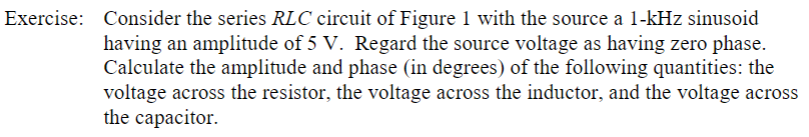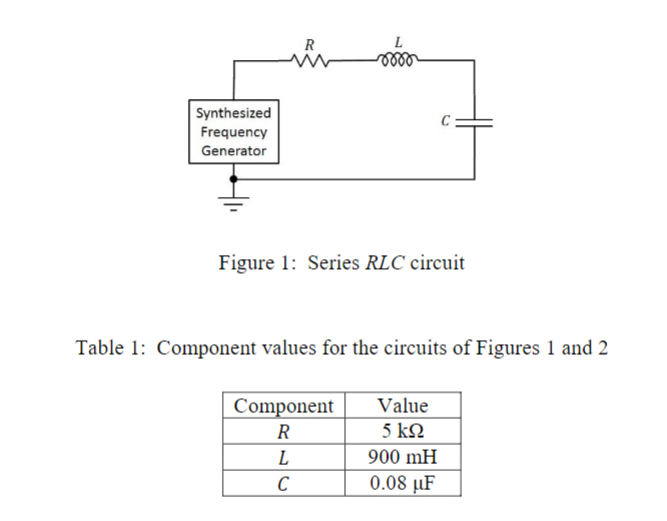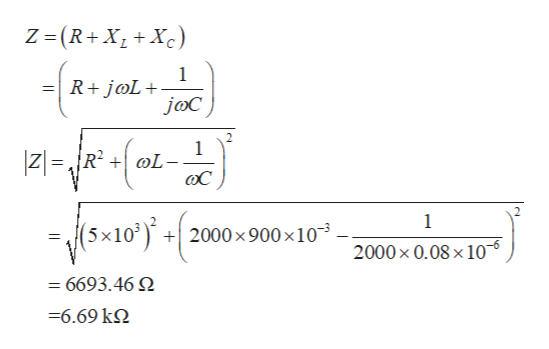# Exercise: Consider the series RLC circuit of Figure 1 with the source a 1-kHz sinusoidhaving an amplitude of 5 V. Regard the source voltage as having zero phase.Calculate the amplitude and phase (in degrees) of the following quantities: thevoltage across the resistor, the voltage across the inductor, and the voltage acrossthe capacitor LRSynthesizedFrequencyGeneratorFigure 1: Series RLC circuitTable 1: Component values for the circuits of Figures 1 and 2ComponentValue5 kΩR900 mHL0.08 uFC

Question
12 viewshelp_outlineImage TranscriptioncloseExercise: Consider the series RLC circuit of Figure 1 with the source a 1-kHz sinusoid having an amplitude of 5 V. Regard the source voltage as having zero phase. Calculate the amplitude and phase (in degrees) of the following quantities: the voltage across the resistor, the voltage across the inductor, and the voltage across the capacitor fullscreenhelp_outlineImage TranscriptioncloseL R Synthesized Frequency Generator Figure 1: Series RLC circuit Table 1: Component values for the circuits of Figures 1 and 2 Component Value 5 kΩ R 900 mH L 0.08 uF C fullscreen
check_circle

Step 1

Angular frequency of source:

Step 2

Source voltage is given as

Step 3

The total impedance of...help_outlineImage TranscriptioncloseZ (R+XXc) 1 R+j@L+ joC ZR2 L f5:10 j 1 2000x 900 x10 2000x 0.08 x 10 = 6693.46 -6.69 k2 fullscreen

### Want to see the full answer?

See Solution

#### Want to see this answer and more?

Solutions are written by subject experts who are available 24/7. Questions are typically answered within 1 hour.*

See Solution
*Response times may vary by subject and question.
Tagged in

### Electrical Engineering